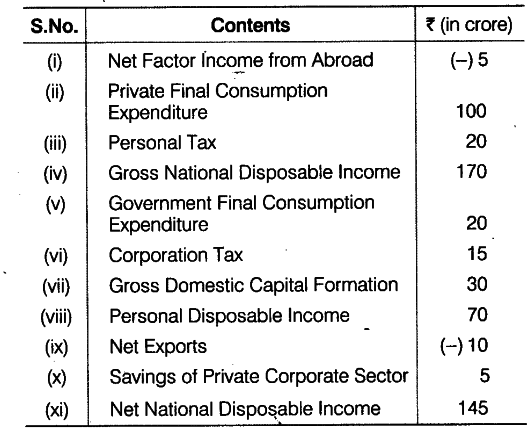# Calculate Net National Product at Market Price

Calculate (a) Net National Product at Market Price and (b) Private income from the following data:Here,
(a) \$NN{{P}_{MP}}\$ = Private Final Consumption Expenditure +Government Final Consumption Expenditure + Gross Domestic Capital Formation + Net Export - Depreciation + NFIA = 100+ 20+ 30+ (- 10)- 25+(-5) = Rs 110 crore
Depreciation = Gross National Disposable Income - Net National Disposable Income = 170-145 = Rs 25 crore
(b) Private Income = Personal Disposable Income + Personal Tax + Corporation Tax

• Savings of Private Corporate Sector = 70 + 20 + 15 + 5 = Rs 110 crore